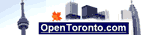# Fahrenheit to Celsius Conversion

Enter °F or °C for conversion:

Select a conversion type:

Rounding options:

 Fahrenheit is a temperature scale named after the German physicist Daniel Gabriel Fahrenheit (16861736), who proposed it in 1724. In this scale, the freezing point of water is 32 degrees Fahrenheit (written "32 °F"), and the boiling point is 212 degrees, placing the boiling and freezing points of water exactly 180 degrees apart. On the Celsius scale, the freezing and boiling points of water are exactly 100 degrees apart, thus the unit of this scale, a degree Fahrenheit, is 5/9 of a degree Celsius. Negative 40 degrees Fahrenheit (-40 °F) is equal to negative 40 degrees Celsius (-40 °C).

Deutsch   English   Espanol   Francais   Italiano   Portuguese

Calculators:
MPG calculator
L/100km fuel calculator
L/100km to MPG calculator
MPG to L/100km conversion
Miles to km conversion
mph to kmh conversion
Gallon to Litre Conversion
Pounds to kilograms conversion
Celsius to Fahrenheit
Fahrenheit to Celsius conversion
Recommended Daily Calorie Calculator
BMI Calculator
Ounces to grams conversion
Yards to mm conversion
Yards to meters Conversion
Feet to meters Conversion
Inches to mm Conversion
Time in Hamilton, Ontario